# Texas Go Math Grade 8 Lesson 3.2 Answer Key Rate of Change and Slope

Refer to our Texas Go Math Grade 8 Answer Key Pdf to score good marks in the exams. Test yourself by practicing the problems from Texas Go Math Grade 8 Lesson 3.2 Answer Key Rate of Change and Slope.

## Texas Go Math Grade 8 Lesson 3.2 Answer Key Rate of Change and Slope

Essential Question
How do you find a rate of change or a slope?

Question 1.
The table shows the approximate height of a football after it is kicked. Tell whether the rates of change are constant or variable. Find the rates of change:
The rates of change are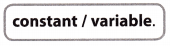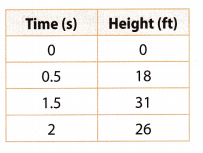Input variable: Time (s) Identify the input and output variables
Output variable: Height (ft)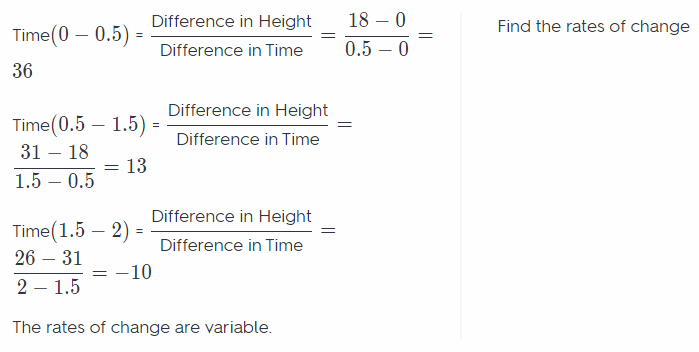Using Graphs to Find Rates of Change
You can also use a graph to find rates of change.

The graph shows the distance Nathan bicycled over time. What is Nathan’s rate of change?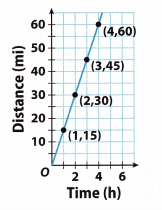A. Find the rate of change from 1 hour to 2 hours.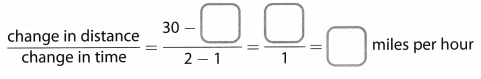B. Find the rate of change from 1 hour to 4 hours.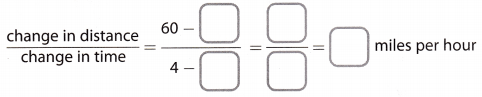C. Find the rate of change from 2 hour to 4 hours.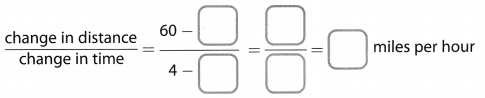D. Recall that the graph of a proportional relationship is a line through the origin. Explain whether the relationship between Nathan’s time and distance is a proportional relationship.

Reflect

Rate of Change and Slope Answer Key Go Math Grade 8 Question 2.
Make a Conjecture Does a proportional relationship have a constant rate of change?
Yes. The following equation holds:
$$\frac{y}{x}$$ = k
Where k is the constant rate of change
Yes

Question 3.
Does it matter what interval you use when you find the rate of change of a proportional relationship? Explain.
No. It does not matter what interval you use as long the order of points remains the same. When y is subtracted from y2, then x1 should be subtracted from x2.

Question 4.
The graph shows the rate at which water is leaking from a tank. The slope of the line gives the leaking rate in gallons per minute.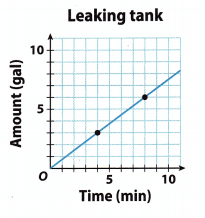Rise = ___________________________
Run = _________________________
Rate of leaking ___ gallon(s) per minute
The two given points are
(4, 3) and (8, 6)
Let x1 = 4, x2 = 8 and y1 = 3, Y2 = 6 We can now calculate the rise, run and slope:
rise = y2 – y1 = 6 – 3 =3
run = x2 – x1 = 8 – 4 = 4
slope = $$\frac{y_{2}-y_{1}}{x_{2}-x_{1}}$$ = $$\frac{3}{4}$$
Rise = 3, Run = 4 and slope = $$\frac{3}{4}$$

Tell whether the rates of change are constant or variable. (example 1)

Question 1.
building measurements ____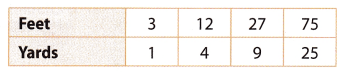(1) First identify the input and output variables
Input variable: Feet, output variable: Yards.
(2) Now find the rates of change.The rates of change are constant: 3 feet per yard.
Constant rate of change

Question 2.
computers sold ____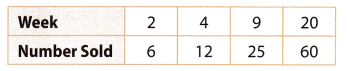Input variable: Week Identify the input and output variables
Output variable: Number soldThe rates of change are variable.

Question 3.
distance an object falls ____Input variable: Week Identify the input and output variables
Output variable: Number soldThe rates of change are variable.
Input variable: Time Identify the input and output variables
Output variable: Distance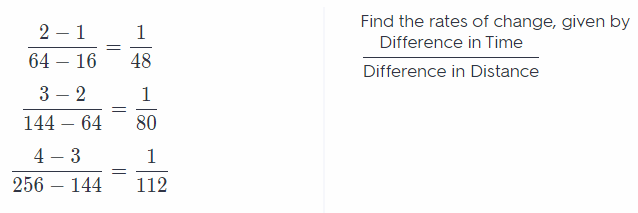The rates of change are variable.

cost of sweaters ____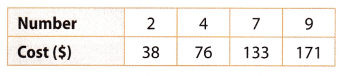(1) First identify the input and output variables.
Input variable: Number, output variable: Cost.

(2) Now find the rates of change.The rates of change are constant: 19 dollars per sweater.
The rates of change are constant.

Erica walks to her friend Philip’s house. The graph shows Erica’s distance from home over time. (Explore Activity)

Question 5.
Find the rate of change from 1 minute to 2 minutes.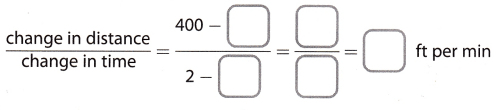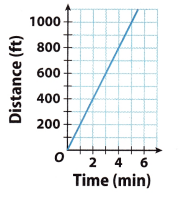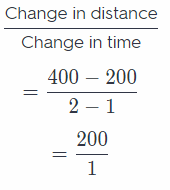Find the rate of change from 1 minute to 2 minutes.
= 200 ft per min

Question 6.
Find the rate of change from 1 minute to 4 minutes. ________Therefore the rate of change from 1 minute to 4 minutes is 200 feet per minute.
200 feet per minute.

Find the slope of each line. (Example 2)

Question 7.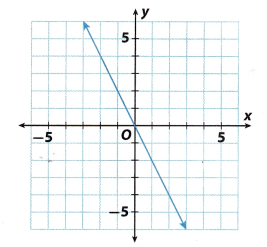Slope = ____
Rise is the difference in values represented by the axis
Rise = 4 – 0 = 4

Run = -2 – 0 = -2 Run is the difference in values represented by the x-axis

Slope = $$\frac{4}{-2}$$ = -2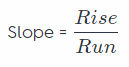Go Math 8th Grade Slope and Rate of Change Answer Key Question 8.Slope = ____
Rise = 3 – 0 = 3 Rise is the difference in values represented by y axis
Run = 2 – 0 = 2 Run is the difference in values represented by x axis
Slope = $$\frac{3}{2}$$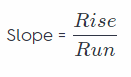Essential Question Check-In

Question 9.
If you know two points on a line, how can you find the rate of change of the variables being graphed?
If the two given points are (x1, y1) and (x2, y2) we can find the slope
slope = $$\frac{y_{2}-y_{1}}{x_{2}-x_{1}}$$
since the slope of a line is the ratio of the change in y-values for a segment of the graph to the corresponding change in x-values.

You can calculate the slope (See inside for more)

Question 10.
Rectangle EFGH is graphed on a coordinate plane with vertices at E(-3, 5), F(6, 2), G(4, -4), and H(-5, -1).

a. Find the slopes of each side.
We are given the vertices of the rectangle EFGH:
E(-3, 5) F(6, 2) G(4, -4) H(-5, -1)

a) In general, the slope of a line is the ratio of the change in y-values for a segment of the graph to the corresponding change in x-values.
Slope = $$\frac{y_{2}-y_{1}}{x_{2}-x_{1}}$$
Slope of EF: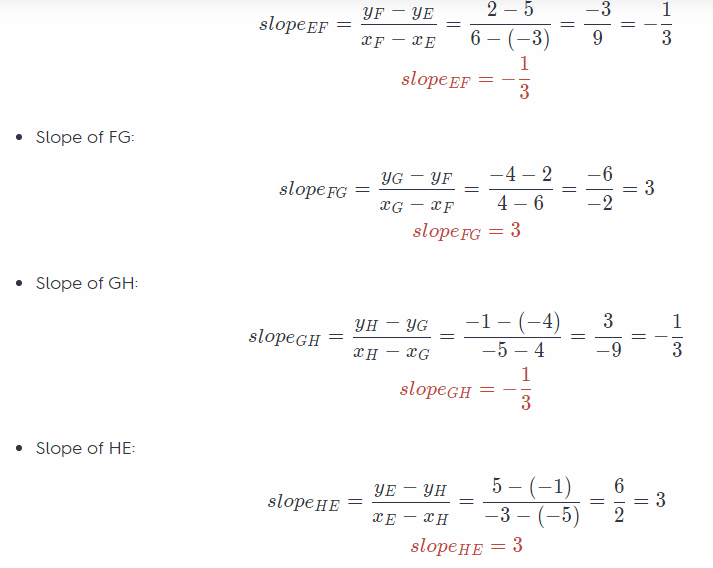b. What do you notice about the slopes of opposite sides?
The slopes of opposite sides are equal
slopeEF = slopeGH = – $$\frac{1}{3}$$
SlopeFG = slopeHE = 3

The slopes of adjacent sides are negative reciprocals of each other (3 and –$$\frac{1}{3}$$)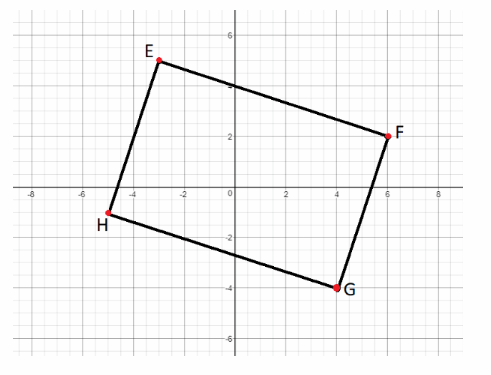A bicyclist started riding at 8:00 a.m The diagram below shows the distance the bicyclist had traveled at different times. What was the bicyclist’s average rate of speed in miles per hour?We are given that the bicyclist rides the first 4.5 miles for 18 minutes and the other 7.5 miles for 30 minutes. To find the rate of speed in miles/hour. First, we need to convert minutes into hours (divide by 60 since 1 hour has 60 minutes).
• Convert 18 minutes into hours:• Convert 30 minutes into hours:The rate of change for the first 0.3 hours:
$$\frac{4.5}{0.3}$$ = $$\frac{45}{3}$$ = 15
The rate of change for the next 0.5 hours:
$$\frac{7.5}{0.5}$$ = $$\frac{75}{5}$$ = 15
In conclusion, the bicyclist’s average rate of speed is 15 miles per hour.

Question 12.
Multistep A line passes through (6, 3), (8,4), and (n, -2). Find the value of n.
Using the first two points:
(x1, y1) = (6, 3) and (x2, y2) = (8, 4) find the slope of the line.
Slope = $$\frac{y_{2}-y_{1}}{x_{2}-x_{1}}$$ = $$\frac{4-3}{8-6}$$ = $$\frac{1}{2}$$
Now use any of the first two points ant the last point to find the value of n
by using the definition of a slope of the line:
Slope = $$\frac{1}{2}$$ = $$\frac{4-(-2)}{8-n}$$ = $$\frac{6}{8-n}$$
$$\frac{6}{12}$$ = $$\frac{6}{8-n}$$
Multiply the numerator and denominator of the left side by 6
12 = 8 – n
n = -4

Go Math Grade 8 Answers 3.2 Rate of Change and Slope Question 13.
A large container holds 5 gallons of water. It begins leaking at a constant rate. After 10 minutes, the container has 3 gallons of water left.
a. At what rate is the water leaking?
At 0 minutes, there are 5 gallons of water. At 10 minutes, there are 3 gallons of water. GivenRate of = $$\frac{5-3}{0-10}$$ = $$\frac{2}{-10}$$ = –$$\frac{1}{5}$$ = -0.2
The rate of water leakage is $1$ gallon every $5$ minute or $0.2$ gallon per min

b. After how many minutes will the container be empty?
No of minutes = = 25
$$\frac{5}{0.2}$$ = 25 The number of minutes is determined by dividing the total volume of container by rate of water leakage.
It will take $25$ minutes for the container to be empty.

Question 14.
Critique Reasoning Billy found the slope of the line through the points (2, 5) and (-2, -5) using the equation $$\frac{2-(-2)}{5-(-5)}$$ = $$\frac{2}{5}$$. What mistake did he make?
By definition, the slope is the change in y-values (rise) for a segment of the graph to the corresponding change in
Slope = $$\frac{y_{2}-y_{1}}{x_{2}-x_{1}}$$
Meanwhile, Billy wrote it as $$\frac{x_{-} 2-x_{-} 1}{y_{2}-y_{1}}$$
So the real slope is $$\frac{5}{2}$$
Billy got the reciprocal of the slope.

Lesson 3.2 Rate of Change and Slope Reteach Answer Key Question 15.
Multiple Representations Graph parallelogram ABCD on a coordinate plane with vertices at A(3, 4), 8(6, 1), C(0, -2), and D(-3, 1).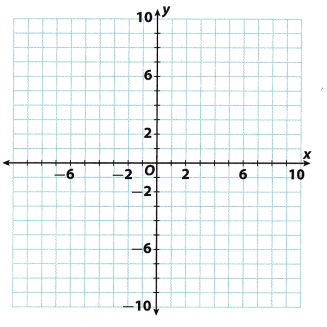We are given the vertices of the parallelogram ABCD:
Ä(3, 4)
B(6, 1)
C(0, -2)
D(-3, 1)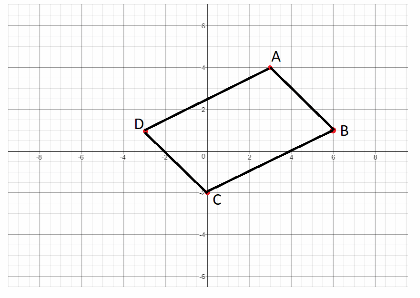a. Find the slope of each side.
In general, the slope of a line is the ratio of the change in y-values for a segment of the graph to the corresponding change in x-values.
slope = $$\frac{y_{2}-y_{1}}{x_{2}-x_{1}}$$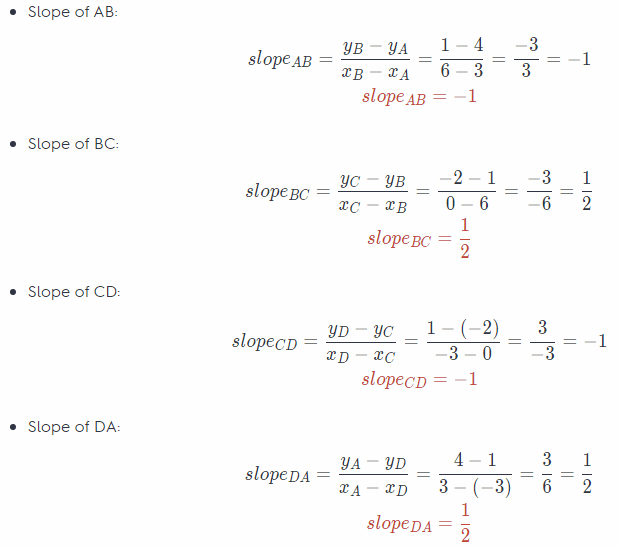b. What do you notice about the slopes?
The slopes of opposite sides are equal.
slopeAB = slopeCD = – 1
slopeBC = SlopeDA = $$\frac{1}{2}$$

c. Draw another parallelogram on the coordinate plane. Do the slopes have the same characteristics?
If we calculate the slopes of the sides of the parallelogram shown below in the same way as calculated above,
we will see that they have the same characteristics.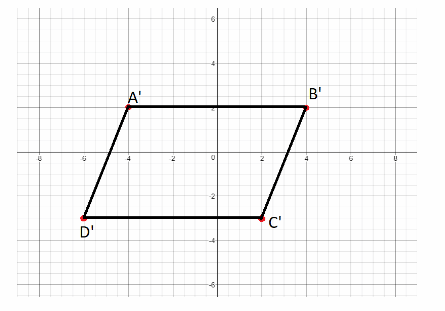Texas Go Math Grade 8 Lesson 3.2 H.O.T. Focus On Higher Order Thinking Answer Key

Question 16.
Communicate Mathematical Ideas Ben and Phoebe are finding the slope of a line. Ben chose two points on the line and used them to find the slope. Phoebe used two different points to find the slope. Did they get the same answer? Explain.
Yes, they did. The slope of a line can be calculated using every two points on the line, since it is the same lane it has a unique (singular) slope

Yes, the slope is the same for every two points.

Lesson 3.2 Rate of Change and Slope Answer Key Question 17.
Analyze Relationships Two lines pass through the origin. The lines have slopes that are opposites. Compare and contrast the lines.